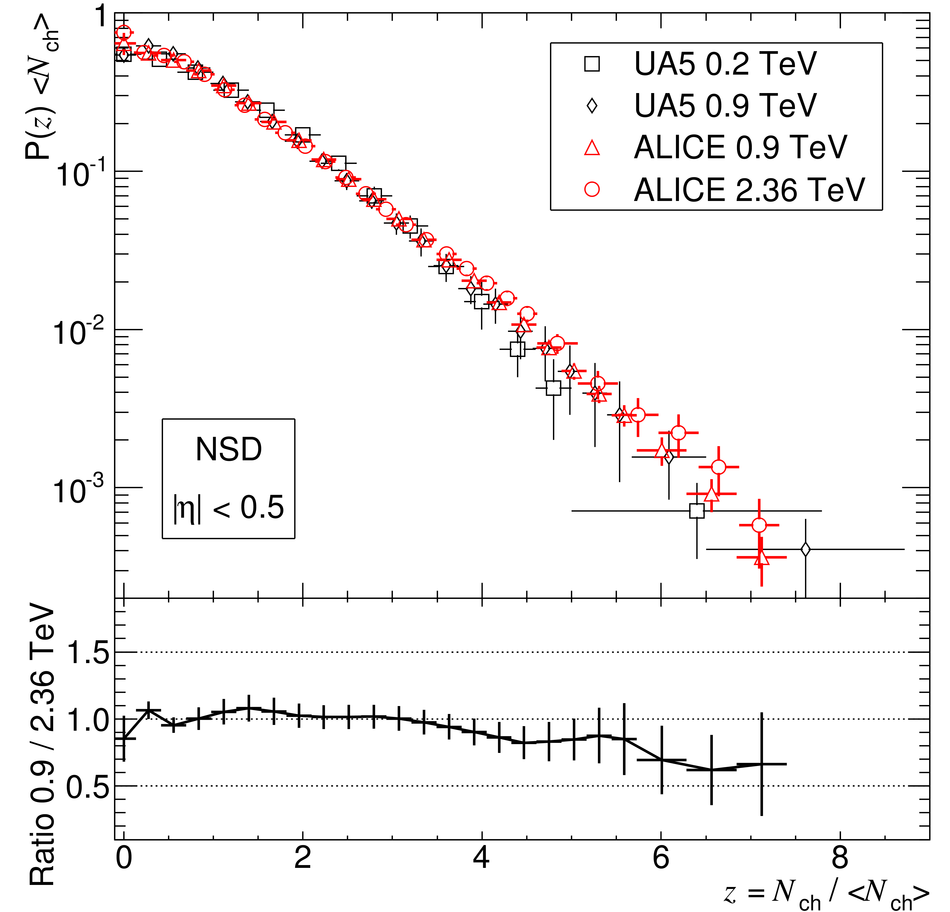# Figure 12

 Comparison of multiplicity distributions in KNO variables measured by UA5 [18,19] in proton-antiproton collisions at $\sqrt{s} = 0.2~$TeV and $0.9~$TeV, and by ALICE at $\sqrt{s} = 0.9~$TeV and $2.36~$TeV, for NSD events in |$\eta$| < 0.5. In the lower part the ratio between ALICE measurements at 0.9 TeV and 2.36 TeV is shown. The error bars represent the combined statistical and systematic uncertainties.